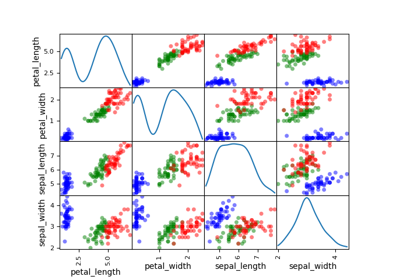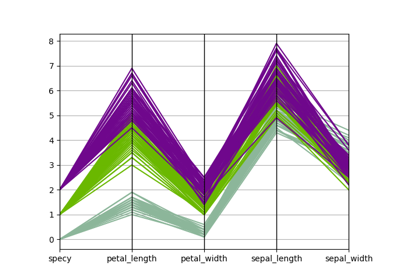gemseo / post / dataset

# parallel_coordinates module¶

Draw parallel coordinates from a Dataset.

The ParallelCoordinates class implements the parallel coordinates plot, a.k.a. cowebplot, which is a way to visualize $$n$$ samples of a high-dimensional vector

$x=(x_1,x_2,\ldots,x_d)\in\mathbb{R}^d$

in a 2D referential by representing each sample

$x^{(i)}=(x_1^{(i)},x_2^{(i)},\ldots,x_d^{(i)})$

as a piece-wise line where the x-values of the nodes from left to right are the values of $$x_1$$, $$x_2$$, … and $$x_d^{(i)}$$.

A variable name is required by the DatasetPlot.execute() method by means of the classifier keyword in order to color the curves according to the value of the variable name. This is useful when the data is labeled or when we are looking for the samples for which the classifier value is comprised in some interval specified by the lower and upper arguments (default values are set to -inf and inf respectively). In the latter case, the color scale is composed of only two values: one for the samples positively classified and one for the others.

class gemseo.post.dataset.parallel_coordinates.ParallelCoordinates(dataset, classifier, lower=-inf, upper=inf, **kwargs)[source]

Bases: DatasetPlot

Parallel coordinates.

Parameters:
• dataset (Dataset) – The dataset containing the data to plot.

• classifier (str) – The name of the variable to group the data.

• lower (float) –

The lower bound of the cluster.

By default it is set to -inf.

• upper (float) –

The upper bound of the cluster.

By default it is set to inf.

• **kwargs – The description is missing.

Raises:

ValueError – If the dataset is empty.

execute(save=True, show=False, file_path=None, directory_path=None, file_name=None, file_format=None, properties=None, fig=None, axes=None, **plot_options)

Execute the post-processing.

Parameters:
• save (bool) –

If True, save the plot.

By default it is set to True.

• show (bool) –

If True, display the plot.

By default it is set to False.

• file_path (str | Path | None) – The path of the file to save the figures. If None, create a file path from directory_path, file_name and file_format.

• directory_path (str | Path | None) – The path of the directory to save the figures. If None, use the current working directory.

• file_name (str | None) – The name of the file to save the figures. If None, use a default one generated by the post-processing.

• file_format (str | None) – A file format, e.g. ‘png’, ‘pdf’, ‘svg’, … If None, use a default file extension.

• properties (Mapping[str, DatasetPlotPropertyType] | None) – The general properties of a DatasetPlot.

• fig (None | Figure) – The figure to plot the data. If None, create a new one.

• axes (None | Axes) – The axes to plot the data. If None, create new ones.

• **plot_options – The options of the current class inheriting from DatasetPlot.

Returns:

The figures.

Raises:

AttributeError – When the name of a property is not the name of an attribute.

Return type:

list[Figure]

color: str | list[str]

The color(s) for the series.

If empty, use a default one.

colormap: str

The color map.

dataset: Dataset

The dataset to be plotted.

fig_size: tuple[float, float]

The figure size.

property fig_size_x: float

The x-component of figure size.

property fig_size_y: float

The y-component of figure size.

font_size: int

The font size.

property labels: Mapping[str, str]

The labels of the variables.

legend_location: str

The location of the legend.

linestyle: str | list[str]

The line style(s) for the series.

If empty, use a default one.

marker: str | list[str]

The marker(s) for the series.

If empty, use a default one.

property output_files: list[str]

The paths to the output files.

title: str

The title of the plot.

xlabel: str

The label for the x-axis.

xmax: float | None

The maximum value on the x-axis.”.

If None, compute it from data.

xmin: float | None

The minimum value on the x-axis.

If None, compute it from data.

ylabel: str

The label for the y-axis.

ymax: float | None

The maximum value on the y-axis.

If None, compute it from data.

ymin: float | None

The minimum value on the y-axis.

If None, compute it from data.

zlabel: str

The label for the z-axis.

zmax: float | None

The maximum value on the z-axis.

If None, compute it from data.

zmin: float | None

The minimum value on the z-axis.

If None, compute it from data.

## Examples using ParallelCoordinates¶Iris dataset

Iris datasetParallel coordinates

Parallel coordinates